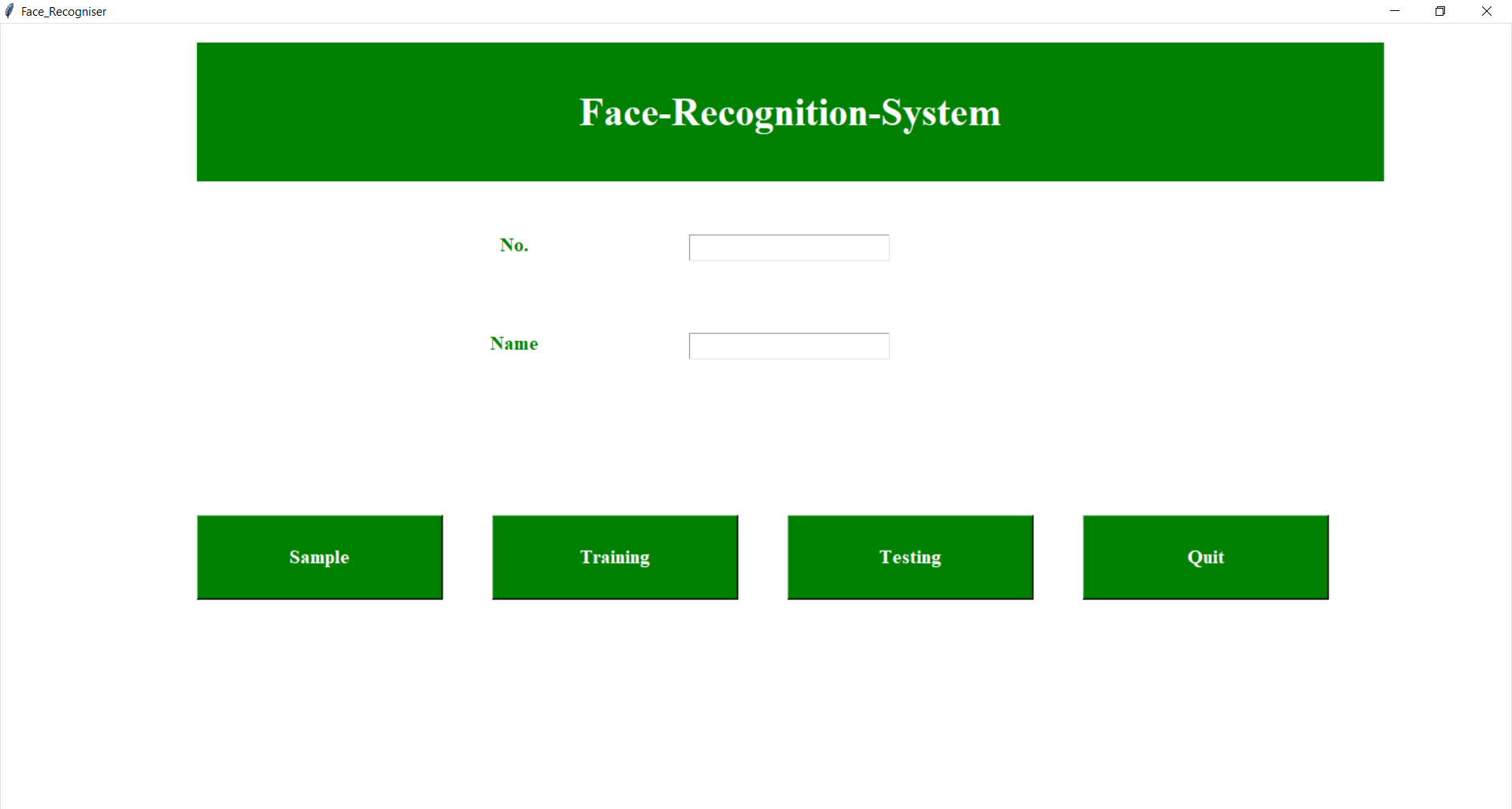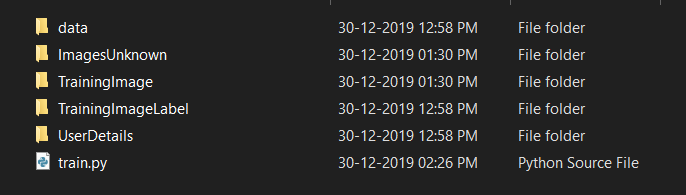Related Articles

# Python | Face recognition using GUI

• Difficulty Level : Medium
• Last Updated : 01 Sep, 2021

In this article, a fairly simple way is mentioned to implement facial recognition system using Python and OpenCV module along with the explanation of the code step by step in the comments.
Before starting we need to install some libraries in order to implement the code. Below you will see the usage of the library along with the code to install it:

• OpenCV:
OpenCV (Open Source Computer Vision Library) is an open-source computer vision and machine learning software library. which is built to provide a common infrastructure for machine learning algorithms and computer vision. It has thousands of optimized algorithms which can be used different purposes like detecting and recognizing faces, identifying objects and many more. We need it to take pictures using our webcam and some manipulation needed to be done in the image.
To install the library you need to install pip in your system after that you can follow the steps in command prompt:
Step 1: pip install opencv-python
Step 2: pip install opencv-contrib-python
• NumPy:
NumPy is the fundamental package for scientific computing in Python which provides a multidimensional array object other mathematical operations can be performed using this but simply speaking we just need it to convert our images into some form of an array so that we can store the model that has been trained.
To install the library you can type a simple line of code in your command shell:
pip install numpy
Haar Cascade is basically a classifier which is used to detect the objects for which it has been trained for, from the source. The result is an XML file which stores the trained result. If said simply the Haar Cascade is trained by superimposing the positive image over a set of negative images. The training requires a high spec system and a good internet connection and thousands of training images that is why it is carried out in the server. For increasing the efficiency of the results they use high-quality images and increase the number of stages for which the classifier is trained. We need haar cascade frontal face recognizer to detect the face from our webcam.
• Python GUI (tkinter):
Tkinter is a simple GUI module used for implementing fairly simple GUI and helps us to interact with code in a simple way. Though for understanding the code its not important for you to know how it works.
Python GUI – tkinter

Code: Python implementing to recognize face using GUI

## Python3

 `# importing libraries``import` `tkinter as tk``from` `tkinter ``import` `Message, Text``import` `cv2``import` `os``import` `shutil``import` `csv``import` `numpy as np``from` `PIL ``import` `Image, ImageTk``import` `pandas as pd``import` `datetime``import` `time``import` `tkinter.ttk as ttk``import` `tkinter.font as font``from` `pathlib ``import` `Path` `window ``=` `tk.Tk()``window.title(``"Face_Recogniser"``)``window.configure(background ``=``'white'``)``window.grid_rowconfigure(``0``, weight ``=` `1``)``window.grid_columnconfigure(``0``, weight ``=` `1``)``message ``=` `tk.Label(``    ``window, text ``=``"Face-Recognition-System"``,``    ``bg ``=``"green"``, fg ``=` `"white"``, width ``=` `50``,``    ``height ``=` `3``, font ``=` `(``'times'``, ``30``, ``'bold'``))``    ` `message.place(x ``=` `200``, y ``=` `20``)` `lbl ``=` `tk.Label(window, text ``=` `"No."``,``width ``=` `20``, height ``=` `2``, fg ``=``"green"``,``bg ``=` `"white"``, font ``=` `(``'times'``, ``15``, ``' bold '``) )``lbl.place(x ``=` `400``, y ``=` `200``)` `txt ``=` `tk.Entry(window,``width ``=` `20``, bg ``=``"white"``,``fg ``=``"green"``, font ``=` `(``'times'``, ``15``, ``' bold '``))``txt.place(x ``=` `700``, y ``=` `215``)` `lbl2 ``=` `tk.Label(window, text ``=``"Name"``,``width ``=` `20``, fg ``=``"green"``, bg ``=``"white"``,``height ``=` `2``, font ``=``(``'times'``, ``15``, ``' bold '``))``lbl2.place(x ``=` `400``, y ``=` `300``)` `txt2 ``=` `tk.Entry(window, width ``=` `20``,``bg ``=``"white"``, fg ``=``"green"``,``font ``=` `(``'times'``, ``15``, ``' bold '``)  )``txt2.place(x ``=` `700``, y ``=` `315``)` `# The function below is used for checking``# whether the text below is number or not ?  ``def` `is_number(s):``    ``try``:``        ``float``(s)``        ``return` `True``    ``except` `ValueError:``        ``pass`` ` `    ``try``:``        ``import` `unicodedata``        ``unicodedata.numeric(s)``        ``return` `True``    ``except` `(TypeError, ValueError):``        ``pass`` ` `    ``return` `False``# Take Images is a function used for creating``# the sample of the images which is used for``# training the model. It takes 60 Images of``# every new user. ``def` `TakeImages():       ``    ` `    ``# Both ID and Name is used for recognising the Image``    ``Id` `=``(txt.get())``    ``name ``=``(txt2.get())``    ` `    ``# Checking if the ID is numeric and name is Alphabetical``    ``if``(is_number(``Id``) ``and` `name.isalpha()):``        ``# Opening the primary camera if you want to access``        ``# the secondary camera you can mention the number``        ``# as 1 inside the parenthesis``        ``cam ``=` `cv2.VideoCapture(``0``)``        ``# Specifying the path to haarcascade file``        ``harcascadePath ``=` `"data\haarcascade_frontalface_default.xml"``        ``# Creating the classier based on the haarcascade file.``        ``detector ``=` `cv2.CascadeClassifier(harcascadePath)``        ``# Initializing the sample number(No. of images) as 0``        ``sampleNum ``=` `0``        ``while``(``True``):``            ``# Reading the video captures by camera frame by frame``            ``ret, img ``=` `cam.read()``            ``# Converting the image into grayscale as most of``            ``# the the processing is done in gray scale format``            ``gray ``=` `cv2.cvtColor(img, cv2.COLOR_BGR2GRAY)``            ` `            ``# It converts the images in different sizes``            ``# (decreases by 1.3 times) and 5 specifies the``            ``# number of times scaling happens``            ``faces ``=` `detector.detectMultiScale(gray, ``1.3``, ``5``)``            ` `            ``# For creating a rectangle around the image``            ``for` `(x, y, w, h) ``in` `faces:``                ``# Specifying the coordinates of the image as well``                ``# as color and thickness of the rectangle.      ``                ``# incrementing sample number for each image``                ``cv2.rectangle(img, (x, y), (``                    ``x ``+` `w, y ``+` `h), (``255``, ``0``, ``0``), ``2``)``                ``sampleNum ``=` `sampleNum ``+` `1``                ``# saving the captured face in the dataset folder``                ``# TrainingImage as the image needs to be trained``                ``# are saved in this folder``                ``cv2.imwrite(``                    ``"TrainingImage\ "``+``name ``+``"."``+``Id` `+``'.'``+` `str``(``                        ``sampleNum) ``+` `".jpg"``, gray[y:y ``+` `h, x:x ``+` `w])``                ``# display the frame that has been captured``                ``# and drawn rectangle around it.``                ``cv2.imshow(``'frame'``, img)``            ``# wait for 100 milliseconds``            ``if` `cv2.waitKey(``100``) & ``0xFF` `=``=` `ord``(``'q'``):``                ``break``            ``# break if the sample number is more than 60``            ``elif` `sampleNum>``60``:``                ``break``        ``# releasing the resources``        ``cam.release()``        ``# closing all the windows``        ``cv2.destroyAllWindows()``        ``# Displaying message for the user``        ``res ``=` `"Images Saved for ID : "` `+` `Id` `+``" Name : "``+` `name ``        ``# Creating the entry for the user in a csv file``        ``row ``=` `[``Id``, name]``        ``with ``open``(``'UserDetails\UserDetails.csv'``, ``'a+'``) as csvFile:``            ``writer ``=` `csv.writer(csvFile)``            ``# Entry of the row in csv file``            ``writer.writerow(row)``        ``csvFile.close()``        ``message.configure(text ``=` `res)``    ``else``:``        ``if``(is_number(``Id``)):``            ``res ``=` `"Enter Alphabetical Name"``            ``message.configure(text ``=` `res)``        ``if``(name.isalpha()):``            ``res ``=` `"Enter Numeric Id"``            ``message.configure(text ``=` `res)``            ` `# Training the images saved in training image folder   ``def` `TrainImages():``    ``# Local Binary Pattern Histogram is an Face Recognizer``    ``# algorithm inside OpenCV module used for training the image dataset``    ``recognizer ``=` `cv2.face.LBPHFaceRecognizer_create() ``    ``# Specifying the path for HaarCascade file``    ``harcascadePath ``=` `"data\haarcascade_frontalface_default.xml"``    ``# creating detector for faces``    ``detector ``=` `cv2.CascadeClassifier(harcascadePath)``    ``# Saving the detected faces in variables``    ``faces, ``Id` `=` `getImagesAndLabels(``"TrainingImage"``)``    ``# Saving the trained faces and their respective ID's``    ``# in a model named as "trainner.yml".``    ``recognizer.train(faces, np.array(``Id``))    ``    ``recognizer.save(``"TrainingImageLabel\Trainner.yml"``)``    ``# Displaying the message``    ``res ``=` `"Image Trained"``    ``message.configure(text ``=` `res)` `def` `getImagesAndLabels(path):``    ``# get the path of all the files in the folder``    ``imagePaths ``=``[os.path.join(path, f) ``for` `f ``in` `os.listdir(path)]``    ``faces ``=``[]``    ``# creating empty ID list``    ``Ids ``=``[]``    ``# now looping through all the image paths and loading the``    ``# Ids and the images saved in the folder``    ``for` `imagePath ``in` `imagePaths:``        ``# loading the image and converting it to gray scale``        ``pilImage ``=` `Image.``open``(imagePath).convert(``'L'``)``        ``# Now we are converting the PIL image into numpy array``        ``imageNp ``=` `np.array(pilImage, ``'uint8'``)``        ``# getting the Id from the image``        ``Id` `=` `int``(os.path.split(imagePath)[``-``1``].split(``"."``)[``1``])``        ``# extract the face from the training image sample``        ``faces.append(imageNp)``        ``Ids.append(``Id``)       ``    ``return` `faces, Ids``# For testing phase``def` `TrackImages():``    ``recognizer ``=` `cv2.face.LBPHFaceRecognizer_create()``    ``# Reading the trained model``    ``recognizer.read(``"TrainingImageLabel\Trainner.yml"``)``    ``harcascadePath ``=` `"data\haarcascade_frontalface_default.xml"``    ``faceCascade ``=` `cv2.CascadeClassifier(harcascadePath)``    ``# getting the name from "userdetails.csv"``    ``df ``=` `pd.read_csv(``"UserDetails\UserDetails.csv"``) ``    ``cam ``=` `cv2.VideoCapture(``0``)``    ``font ``=` `cv2.FONT_HERSHEY_SIMPLEX       ``    ``while` `True``:``        ``ret, im ``=` `cam.read()``        ``gray ``=` `cv2.cvtColor(im, cv2.COLOR_BGR2GRAY)``        ``faces ``=` `faceCascade.detectMultiScale(gray, ``1.2``, ``5``)   ``        ``for``(x, y, w, h) ``in` `faces:``            ``cv2.rectangle(im, (x, y), (x ``+` `w, y ``+` `h), (``225``, ``0``, ``0``), ``2``)``            ``Id``, conf ``=` `recognizer.predict(gray[y:y ``+` `h, x:x ``+` `w])                                  ``            ``if``(conf < ``50``):``                ``aa ``=` `df.loc[df[``'Id'``] ``=``=` `Id``][``'Name'``].values``                ``tt ``=` `str``(``Id``)``+``"-"``+``aa   ``            ``else``:``                ``Id` `=``'Unknown'`               `                ``tt ``=` `str``(``Id``) ``            ``if``(conf > ``75``):``                ``noOfFile ``=` `len``(os.listdir(``"ImagesUnknown"``))``+``1``                ``cv2.imwrite(``"ImagesUnknown\Image"``+``                ``str``(noOfFile) ``+` `".jpg"``, im[y:y ``+` `h, x:x ``+` `w])           ``            ``cv2.putText(im, ``str``(tt), (x, y ``+` `h),``            ``font, ``1``, (``255``, ``255``, ``255``), ``2``)       ``        ``cv2.imshow(``'im'``, im)``        ``if` `(cv2.waitKey(``1``)``=``=` `ord``(``'q'``)):``            ``break``    ``cam.release()``    ``cv2.destroyAllWindows()``  ` `  ` `takeImg ``=` `tk.Button(window, text ``=``"Sample"``,``command ``=` `TakeImages, fg ``=``"white"``, bg ``=``"green"``,``width ``=` `20``, height ``=` `3``, activebackground ``=` `"Red"``,``font ``=``(``'times'``, ``15``, ``' bold '``))``takeImg.place(x ``=` `200``, y ``=` `500``)``trainImg ``=` `tk.Button(window, text ``=``"Training"``,``command ``=` `TrainImages, fg ``=``"white"``, bg ``=``"green"``,``width ``=` `20``, height ``=` `3``, activebackground ``=` `"Red"``,``font ``=``(``'times'``, ``15``, ``' bold '``))``trainImg.place(x ``=` `500``, y ``=` `500``)``trackImg ``=` `tk.Button(window, text ``=``"Testing"``,``command ``=` `TrackImages, fg ``=``"white"``, bg ``=``"green"``,``width ``=` `20``, height ``=` `3``, activebackground ``=` `"Red"``,``font ``=``(``'times'``, ``15``, ``' bold '``))``trackImg.place(x ``=` `800``, y ``=` `500``)``quitWindow ``=` `tk.Button(window, text ``=``"Quit"``,``command ``=` `window.destroy, fg ``=``"white"``, bg ``=``"green"``,``width ``=` `20``, height ``=` `3``, activebackground ``=` `"Red"``,``font ``=``(``'times'``, ``15``, ``' bold '``))``quitWindow.place(x ``=` `1100``, y ``=` `500``)` ` ` `window.mainloop()`

Sample Image of the GUI:Folder Structure For reference :Attention reader! Don’t stop learning now. Get hold of all the important Machine Learning Concepts with the Machine Learning Foundation Course at a student-friendly price and become industry ready.

My Personal Notes arrow_drop_up